# SmallPT —— 99 行代码光线追踪解析

Rhythm & Hues Studios 公司的程序员 Kevin Beason 曾于 2010 年编写过一个名为 SmallPT 的 C++ 程序，仅包含 99 行代码，即实现了最简单的光线追踪效果。此程序可视为光追算法的可运行最小集，是初学者学习和理解光追原理的极佳材料，其运行结果如下图所示。作为一个门外汉，我花了好几个晚上研究这 99 行代码，并在这个极好的 PPT 的帮助下，总算基本弄明白了其运行的原理。不妨记录下来：

## 渲染方程

\begin{equation}L_o(o)=L_e(o)+\int_\Omega L_i(i)\cdot F(i, o)\cdot \cos\theta\cdot di \end{equation}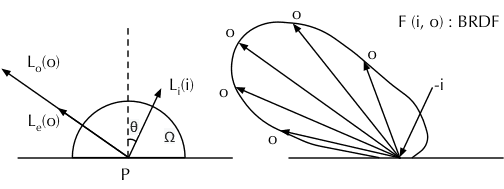1. 此方程描述的问题是：从物体表面上的一点 $P$ 处，射入到观察者眼中的某条光线的强度，是如何确定的。
2. $L_o(o)$ 为射入观察者眼中的光线的颜色，即需要求取的值；$o$ 为出射方向。
3. $L_e(o)$ 为物体表面在点 $P$ 向观察者方向自发射的光线的颜色（灯）。
4. $L_i(i)$ 表示环境入射到点 $P$ 的光的颜色；$i$ 代表入射方向（为计算方便，取真实入射方向的反方向，不影响代表关系，后面也简称为入射方向）。
5. $F(i,o)$ 表示在给定 $i$ 和 $o$ 时，由 $i$ 方向的入射单位光强产生的 $o$ 方向的出射光的强度，此函数与表面的性质有关，又称表面的 BRDF 函数。
6. $\theta$ 表示 $i$ 与表面法线的夹角。
7. $\int_\Omega L_i(i)\cdot F(i, o)\cdot \cos\theta\cdot di$ 整个积分项表示：对半球（不透明材质）或全球（透明介质）内的所有入射方向 $i$ 进行积分，得到的 $o$ 方向的出射光强度。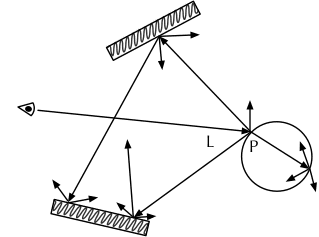## 蒙特卡洛方法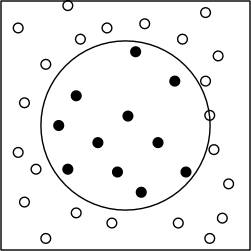\begin{equation}\int_{a}^{b} f(x)\cdot dx \approx \sum_{x=rand(a,b)}^{N} f(x) \cdot \frac{b-a}{N} \end{equation}

1. 左侧表示函数 $f(x)$ 在区间 $[a, b]$ 的积分，即下图中的部分阴影部分面积。
2. 此积分的值，可以这样求取：随机在 $[a, b]$ 区间取值，采样计算 $f(x)$，然后计算所有样本的均值并乘以区间的长度。当取样数量 $N$ 越大，最后的值就越接近真实的积分值。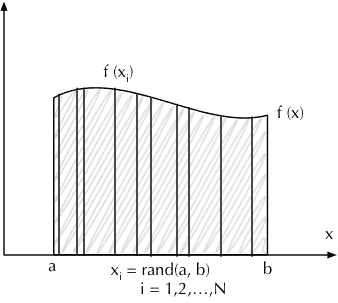\begin{equation}\int_{y_1}^{y_2} \int_{x_1}^{x_2} f(x, y)\cdot dxdy \approx \sum_{ \begin{matrix}x=rand(x_1,x_2) \\ y=rand(y_1,y_2) \end{matrix} }^{N} f(x,y) \cdot \frac{(x_2-x_1)\cdot (y_2-y_1)}{N} \end{equation}

## 光线追踪算法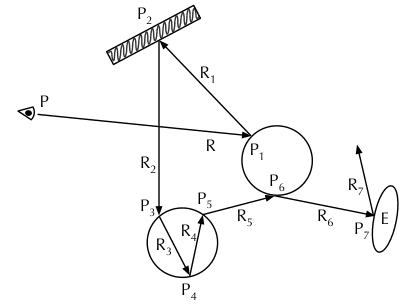1. 从每个像素 $P$ 处发出一条射线 $R$，其方向与入射到相机并产生该像素的光线相反。
2. 求取此射线照射到的物体表面的点 $P_1$，即与场景中物体的交点；如有多个交点，取距离相机最近的那个。如果未求到交点，则返回背景色。
3. $P_1$ 射向 $P$ 的光线强度，为 $P_1$ 本身发射光强度 $E$，加上反射或折射环境光的强度。
1. $E$：如果 $P_1$ 处是光源，则 $E$ 为光源的强度；否则，$E$ 为 0。
2. $R_1$：根据 $P_1$ 处根据表面性质，按概率随机取一次反射的射线 $R_2$，然后重复 2 的步骤，分别递归地求取 $P_2$，$R_3$，$P_3$，$R_4$ ……等等，直到满足一些特定条件停止递归。

## 代码摘录

#include <math.h>   // smallpt, a Path Tracer by Kevin Beason, 2008
#include <stdlib.h> // Make : g++ -O3 -fopenmp smallpt.cpp -o smallpt
#include <stdio.h>  //        Remove "-fopenmp" for g++ version < 4.2
struct Vec {        // Usage: time ./smallpt 5000 && xv image.ppm
double x, y, z;                  // position, also color (r,g,b)
Vec(double x_=0, double y_=0, double z_=0){ x=x_; y=y_; z=z_; }
Vec operator+(const Vec &b) const { return Vec(x+b.x,y+b.y,z+b.z); }
Vec operator-(const Vec &b) const { return Vec(x-b.x,y-b.y,z-b.z); }
Vec operator*(double b) const { return Vec(x*b,y*b,z*b); }
Vec mult(const Vec &b) const { return Vec(x*b.x,y*b.y,z*b.z); }
Vec& norm(){ return *this = *this * (1/sqrt(x*x+y*y+z*z)); }
double dot(const Vec &b) const { return x*b.x+y*b.y+z*b.z; } // cross:
Vec operator%(Vec&b){return Vec(y*b.z-z*b.y,z*b.x-x*b.z,x*b.y-y*b.x);}
};
struct Ray { Vec o, d; Ray(Vec o_, Vec d_) : o(o_), d(d_) {} };
enum Refl_t { DIFF, SPEC, REFR };  // material types, used in radiance()
struct Sphere {
Vec p, e, c;      // position, emission, color
Refl_t refl;      // reflection type (DIFFuse, SPECular, REFRactive)
Sphere(double rad_, Vec p_, Vec e_, Vec c_, Refl_t refl_):
double intersect(const Ray &r) const { // returns distance, 0 if nohit
Vec op = p-r.o; // Solve t^2*d.d + 2*t*(o-p).d + (o-p).(o-p)-R^2 = 0
if (det<0) return 0; else det=sqrt(det);
return (t=b-det)>eps ? t : ((t=b+det)>eps ? t : 0);
}
};
Sphere spheres[] = {//Scene: radius, position, emission, color, material
Sphere(1e5, Vec( 1e5+1,40.8,81.6), Vec(),Vec(.75,.25,.25),DIFF),//Left
Sphere(1e5, Vec(-1e5+99,40.8,81.6),Vec(),Vec(.25,.25,.75),DIFF),//Rght
Sphere(1e5, Vec(50,40.8, 1e5),     Vec(),Vec(.75,.75,.75),DIFF),//Back
Sphere(1e5, Vec(50,40.8,-1e5+170), Vec(),Vec(),           DIFF),//Frnt
Sphere(1e5, Vec(50, 1e5, 81.6),    Vec(),Vec(.75,.75,.75),DIFF),//Botm
Sphere(1e5, Vec(50,-1e5+81.6,81.6),Vec(),Vec(.75,.75,.75),DIFF),//Top
Sphere(16.5,Vec(27,16.5,47),       Vec(),Vec(1,1,1)*.999, SPEC),//Mirr
Sphere(16.5,Vec(73,16.5,78),       Vec(),Vec(1,1,1)*.999, REFR),//Glas
Sphere(600, Vec(50,681.6-.27,81.6),Vec(12,12,12),  Vec(), DIFF) //Lite
};
inline double clamp(double x){ return x<0 ? 0 : x>1 ? 1 : x; }
inline int toInt(double x){ return int(pow(clamp(x),1/2.2)*255+.5); }
inline bool intersect(const Ray &r, double &t, int &id){
double n=sizeof(spheres)/sizeof(Sphere), d, inf=t=1e20;
for(int i=int(n);i--;) if((d=spheres[i].intersect(r))&&d<t){t=d;id=i;}
return t<inf;
}
Vec radiance(const Ray &r, int depth, unsigned short *Xi){
double t;                               // distance to intersection
int id=0;                               // id of intersected object
if (!intersect(r, t, id)) return Vec(); // if miss, return black
const Sphere &obj = spheres[id];        // the hit object
Vec x=r.o+r.d*t, n=(x-obj.p).norm(), nl=n.dot(r.d)<0?n:n*-1, f=obj.c;
double p = f.x>f.y && f.x>f.z ? f.x : f.y>f.z ? f.y : f.z; // max refl
if (++depth>5) if (erand48(Xi)<p) f=f*(1/p); else return obj.e; //R.R.
if (obj.refl == DIFF){                  // Ideal DIFFUSE reflection
double r1=2*M_PI*erand48(Xi), r2=erand48(Xi), r2s=sqrt(r2);
Vec w=nl, u=((fabs(w.x)>.1?Vec(0,1):Vec(1))%w).norm(), v=w%u;
Vec d = (u*cos(r1)*r2s + v*sin(r1)*r2s + w*sqrt(1-r2)).norm();
} else if (obj.refl == SPEC)            // Ideal SPECULAR reflection
Ray reflRay(x, r.d-n*2*n.dot(r.d));     // Ideal dielectric REFRACTION
bool into = n.dot(nl)>0;                // Ray from outside going in?
double nc=1, nt=1.5, nnt=into?nc/nt:nt/nc, ddn=r.d.dot(nl), cos2t;
if ((cos2t=1-nnt*nnt*(1-ddn*ddn))<0)    // Total internal reflection
Vec tdir = (r.d*nnt - n*((into?1:-1)*(ddn*nnt+sqrt(cos2t)))).norm();
double a=nt-nc, b=nt+nc, R0=a*a/(b*b), c = 1-(into?-ddn:tdir.dot(n));
double Re=R0+(1-R0)*c*c*c*c*c,Tr=1-Re,P=.25+.5*Re,RP=Re/P,TP=Tr/(1-P);
return obj.e + f.mult(depth>2 ? (erand48(Xi)<P ? // Russian roulette
}
int main(int argc, char *argv[]){
int w=1024/8, h=768/8, samps = argc==2 ? atoi(argv)/4 : 30;
Ray cam(Vec(50,52,295.6), Vec(0,-0.042612,-1).norm()); // cam pos, dir
Vec cx=Vec(w*.5135/h), cy=(cx%cam.d).norm()*.5135, r, *c=new Vec[w*h];
for (int y=0; y<h; y++){                       // Loop over image rows
fprintf(stderr,"\rRendering (%d spp) %5.2f%%",samps*4,100.*y/(h-1));
for (unsigned short x=0, Xi={0,0,(unsigned short)(y*y*y)}; x<w; x++)
for (int sy=0, i=(h-y-1)*w+x; sy<2; sy++)     // 2x2 subpixel rows
for (int sx=0; sx<2; sx++, r=Vec()){        // 2x2 subpixel cols
for (int s=0; s<samps; s++){
double r1=2*erand48(Xi), dx=r1<1 ? sqrt(r1)-1: 1-sqrt(2-r1);
double r2=2*erand48(Xi), dy=r2<1 ? sqrt(r2)-1: 1-sqrt(2-r2);
Vec d = cx*( ( (sx+.5 + dx)/2 + x)/w - .5) +
cy*( ( (sy+.5 + dy)/2 + y)/h - .5) + cam.d;
} // Camera rays are pushed ^^^^^ forward to start in interior
c[i] = c[i] + Vec(clamp(r.x),clamp(r.y),clamp(r.z))*.25;
}
}
FILE *f = fopen("image.ppm", "w");         // Write image to PPM file.
fprintf(f, "P3\n%d %d\n%d\n", w, h, 255);
for (int i=0; i<w*h; i++)
fprintf(f,"%d %d %d ", toInt(c[i].x), toInt(c[i].y), toInt(c[i].z));
}

## 矢量运算

struct Vec {
double x, y, z;
Vec(double x_=0, double y_=0, double z_=0){ x=x_; y=y_; z=z_; }
Vec operator+(const Vec &b) const { return Vec(x+b.x,y+b.y,z+b.z); }
Vec operator-(const Vec &b) const { return Vec(x-b.x,y-b.y,z-b.z); }
Vec operator*(double b) const { return Vec(x*b,y*b,z*b); }
Vec mult(const Vec &b) const { return Vec(x*b.x,y*b.y,z*b.z); }
Vec& norm(){ return *this = *this * (1/sqrt(x*x+y*y+z*z)); }
double dot(const Vec &b) const { return x*b.x+y*b.y+z*b.z; }
Vec operator%(Vec&b){return Vec(y*b.z-z*b.y,z*b.x-x*b.z,x*b.y-y*b.x);}
};

1. 加法 operator+：$V_1+V_2=(x_1+x_2,y_1+y_2,z_1+z_2)$。
2. 减法 operator-：$V_1-V_2=(x_1-x_2,y_1-y_2,z_1-z_2)$。
3. 乘法 mult()：$mult(V_1, V_2)=(x_1 x_2,y_1 y_2,z_1 z_2)$。这种矢量分量直接相乘的运算通常用于颜色的计算，在三维空间中并无特殊的物理意义。
4. 点乘 dot()：$V_1\cdot V_2 = (x_1 x_2 + y_1 y_2 + z_1 z_2) = \cos\theta$，其中 $\theta$ 为 $V_1$ 与 $V_2$ 的夹角。点乘的结果是一个标量，物理含义是 $V_1$ 在 $V_2$ 方向上的投影。
5. 叉乘 operator%：$V_1\times V_2 = (y_1 z_2 - z_1 y_2, z_1 x_2 - x_1 z_2, x_1 y_2 - y_1 x_2)$，叉乘的结果是一个矢量，物理意义是垂直于 $V_1$ 和 $V_2$ 的矢量（按 $V_1\rightarrow V_2$ 顺序右手螺旋），其长度为 $V_1$ 和 $V_2$ 构成的平行四边形面积。
6. 矢量乘以标量 operator*：$V_1 * b = (x_1 b, y_1 b, z_1 b)$。
7. 归一化 norm()：$norm(V_1) = V_1 * \frac{1}{\sqrt {x_1^2+y_1^2+z_1^2}}$，其物理意义是保持矢量方向不变，将其长度缩放为单位长度 1。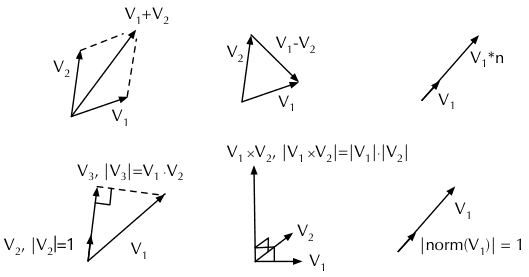## 射线

struct Ray { Vec o, d; Ray(Vec o_, Vec d_) : o(o_), d(d_) {} };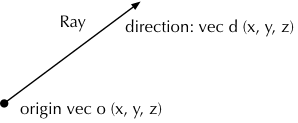## 定义场景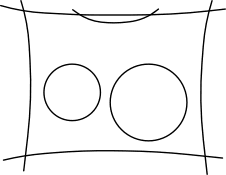### 球体对象的表示

1. 首先枚举出三种表面特性：散射面——用于墙壁，镜面——用于左侧的镜面小球，折射面——用于右侧的玻璃小球。
2. 定义球体，内容包括：
1. 数值类型成员：半径 $rad$；
2. 矢量类型成员：圆心位置 $p$，表面发光强度（颜色）$e$，表面散射颜色 $c$；
3. 表面特性 $refl$；
enum Refl_t { DIFF, SPEC, REFR };  // material types, used in radiance()
struct Sphere {
Vec p, e, c;      // position, emission, color
Refl_t refl;      // reflection type (DIFFuse, SPECular, REFRactive)
Sphere(double rad_, Vec p_, Vec e_, Vec c_, Refl_t refl_):
// ...
};

### 射线与球体相交

Sphere 上定义了 intersect 方法，以求取给定的射线与球体是不是相交。如果相交则返回射线原点与交点的距离。

double intersect(const Ray &r) const { // returns distance, 0 if nohit
Vec op = p-r.o; // Solve t^2*d.d + 2*t*(o-p).d + (o-p).(o-p)-R^2 = 0
if (det<0) return 0; else det=sqrt(det);
return (t=b-det)>eps ? t : ((t=b+det)>eps ? t : 0);
}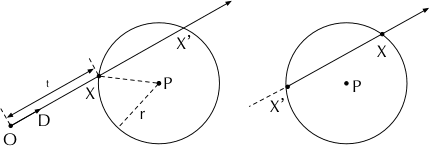1. $X$ 点在射线上，即 $X=O+D*t$。
2. $X$ 点在球面上，即 $X$ 与球心 $P$ 的距离等于球的半径 。即 $|X-P|=r$，也就是 $(X-P)^2=r^2$。

\begin{equation}(O+D*t-P)^2=r^2\end{equation}

\begin{equation}D^2*t+2(O-P)\cdot D*t+(O-P)^2-r^2=0\end{equation}

\begin{equation}x=\frac{-b\pm \sqrt{b^2-4ac}}{2 a}\end{equation}

1. 一元二次方程可能没有实根的，此时射线与球体不相交。这对应着代码中 det<0 的情况，直接返回 0。
2. 一元二次方程可能有两个实根，此时射线（所在的直线）与球体有两个交点。我们需要取的是最小的正根。如果另一个根 $t'$（对应交点为 $X'$）大于 $t$，表明是左图的情况；如果 $t'$ 小于等于 0，表明是右图的情况，这条光线是在介质内的折射光线。

### 使用球体表示场景

1. 左侧墙体，红色；墙体的半径都很大，观者感知不到曲率；墙体的反射类型都是散射，但是颜色有所不同。
2. 右侧墙体，蓝色。
3. 后面墙体，灰色。
4. 前面墙体，黑色。
5. 底部墙体，灰色。
6. 顶部墙体，灰色。
7. 左侧球体，镜面材质。
8. 右侧球体，透明玻璃材质，光线可能折射进入玻璃中继续传播。
9. 顶部的光源，发射出强度为 12 的白光。
Sphere spheres[] = { //Scene: radius, position, emission, color, material
Sphere(1e5, Vec( 1e5+1,40.8,81.6), Vec(),Vec(.75,.25,.25),DIFF),//Left
Sphere(1e5, Vec(-1e5+99,40.8,81.6),Vec(),Vec(.25,.25,.75),DIFF),//Rght
Sphere(1e5, Vec(50,40.8, 1e5),     Vec(),Vec(.75,.75,.75),DIFF),//Back
Sphere(1e5, Vec(50,40.8,-1e5+170), Vec(),Vec(),           DIFF),//Frnt
Sphere(1e5, Vec(50, 1e5, 81.6),    Vec(),Vec(.75,.75,.75),DIFF),//Botm
Sphere(1e5, Vec(50,-1e5+81.6,81.6),Vec(),Vec(.75,.75,.75),DIFF),//Top
Sphere(16.5,Vec(27,16.5,47),       Vec(),Vec(1,1,1)*.999, SPEC),//Mirr
Sphere(16.5,Vec(73,16.5,78),       Vec(),Vec(1,1,1)*.999, REFR),//Glas
Sphere(600, Vec(50,681.6-.27,81.6),Vec(12,12,12),  Vec(), DIFF) //Lite
};

## 准备渲染

### 确定相机和输出图像

int main(int argc, char *argv[]){
int w=1024, h=768, samps = argc==2 ? atoi(argv)/4 : 30;
Ray cam(Vec(50,52,295.6), Vec(0,-0.042612,-1).norm()); // cam pos, dir
Vec cx=Vec(w*.5135/h), cy=(cx%cam.d).norm()*.5135, r, *c=new Vec[w*h];
for (int y=0; y<h; y++){                       // Loop over image rows
fprintf(stderr,"\rRendering (%d spp) %5.2f%%",samps*4,100.*y/(h-1));
for (unsigned short x=0, Xi={0,0,(unsigned short)(y*y*y)}; x<w; x++)
// ... 暂时先省略
}
FILE *f = fopen("image.ppm", "w");         // Write image to PPM file.
fprintf(f, "P3\n%d %d\n%d\n", w, h, 255);
for (int i=0; i<w*h; i++)
fprintf(f,"%d %d %d ", toInt(c[i].x), toInt(c[i].y), toInt(c[i].z));
}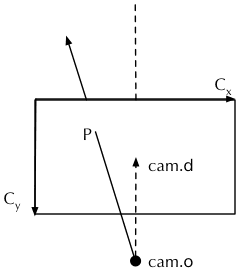PPM 是一种基于文本的图片格式，能够帮助你避免考虑图片文件编码问题，轻易地按像素输出图片。比如以下文本内容就是一张 4x4 的图片。

P3
# feep.ppm
4 4
15
0  0  0    0  0  0    0  0  0   15  0 15
0  0  0    0 15  7    0  0  0    0  0  0
0  0  0    0  0  0    0 15  7    0  0  0
15  0 15    0  0  0    0  0  0    0  0  01. wh：宽度和高度像素数。
2. samps：每个像素采样次数的 1/4。
3. cam：相机描述。
4. cxcy：长度与幕布真实尺寸相同的水平、垂直矢量。
5. c：按像素存储渲染结果的数组。

### 子像素采样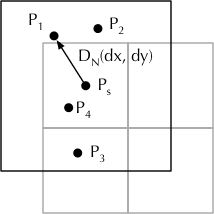\begin{equation}f(x) = \left \{ \begin{matrix} \sqrt{x} -1 \leftarrow x<1 \\ 1-\sqrt{2-x} \leftarrow x>1 \end{matrix} \right. \end{equation}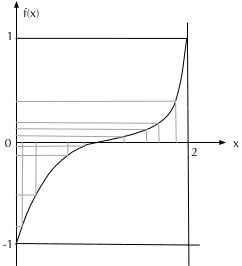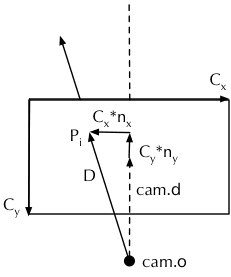for (int sy=0, i=(h-y-1)*w+x; sy<2; sy++)     // 2x2 subpixel rows
for (int sx=0; sx<2; sx++, r=Vec()){        // 2x2 subpixel cols
for (int s=0; s<samps; s++){
double r1=2*erand48(Xi), dx=r1<1 ? sqrt(r1)-1: 1-sqrt(2-r1);
double r2=2*erand48(Xi), dy=r2<1 ? sqrt(r2)-1: 1-sqrt(2-r2);
Vec d = cx*( ( (sx+.5 + dx)/2 + x)/w - .5) +
cy*( ( (sy+.5 + dy)/2 + y)/h - .5) + cam.d;
} // Camera rays are pushed ^^^^^ forward to start in interior
c[i] = c[i] + Vec(clamp(r.x),clamp(r.y),clamp(r.z))*.25;
}

erand48() 函数是 C 标准库提供的随机函数。传入长度为 3 的数组作为随机种子，返回 [0, 1] 区间的双精度浮点数，同时改写传入的随机种子，便于下一次调用 erand() 时传入（以获取一个不同的结果）。

• i：为当前像素在 c 中的索引。
• r：通过光追计算出的子像素的颜色。
• sxsy：子像素索引，为 0 或 1。
• r1r2：滤波用自变量，在 [0, 2] 区间随机取得。
• dxdy：滤波后的随机自变量，分布在 [-1, 1] 区间内。
• d ：从相机原点指向子像素位置的矢量。

## 光线追踪

Vec radiance(const Ray &r, int depth, unsigned short *Xi){
double t;                               // distance to intersection
int id=0;                               // id of intersected object
if (!intersect(r, t, id)) return Vec(); // if miss, return black
const Sphere &obj = spheres[id];        // the hit object
Vec x=r.o+r.d*t, n=(x-obj.p).norm(), nl=n.dot(r.d)<0?n:n*-1, f=obj.c;
double p = f.x>f.y && f.x>f.z ? f.x : f.y>f.z ? f.y : f.z; // max refl
if (++depth>5) if (erand48(Xi)<p) f=f*(1/p); else return obj.e; //R.R.
if (obj.refl == DIFF){                  // Ideal DIFFUSE reflection
// ...
} else if (obj.refl == SPEC)            // Ideal SPECULAR reflection
// ...
// ...                                   // Ideal dielectric REFRACTION
}

### 求取交点和递归结束条件

inline bool intersect(const Ray &r, double &t, int &id){
double n=sizeof(spheres) /sizeof(Sphere), d, inf=t=1e20;
for(int i=int(n);i--;) if((d=spheres[i].intersect(r))&&d<t){t=d;id=i;}
return t<inf;
}

1. 放弃反射光的贡献，直接返回物体的出射光——虽然大部分时候是 0（递归的结束条件 2）。
2. 继续追踪光线。

• t：交点距离相机原点的距离。
• id：相交的球体在 spheres 数组中的索引。
• obj：相交的球体 Sphere 对象。
• x：交点的位置。
• n：球体在交点处的归一化的法线（从球心射向表面）。
• nl：与反射/折射上下文契合的归一化的法线：如果射线在球体外部，nln 相同；如果射线在球体内部（折射），nln 相反。

### 散射（漫反射）

1. 确定互相正交的三个归一化矢量 $U$，$V$ 和 $W$，其中 $W$ 为反射面的法线。
2. 在区间 $[0, 1]$ 中随机确定一个值 $r_2$，并使 $\sqrt{1-r_2^2}$ 作为反射光线 $D$ 在法线 $W$ 方向上投影的长度，使 $\sqrt{r_2}$ 也就是 $r_2 s$ 成为反射光线在表面上投影的长度。
3. 在区间 $[0, 2\pi]$ 中随机确定一个角度值 $r1$，使之成为反射光点 $D$ 在反射表面（即 $UV$ 平面）的投影与 $U$ 轴的交角。
4. 极坐标公式转笛卡尔坐标，$U\ast\cos(r_1)\ast r_2s+V\ast\sin(r_1)\ast r_2s+W\ast \sqrt{1-r_2s^2}$ 计算出在半球面的随机矢量，再归一化得到反射矢量 $D$。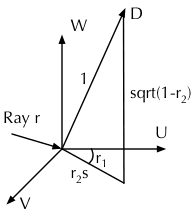if (obj.refl == DIFF){                  // Ideal DIFFUSE reflection
double r1=2*M_PI*erand48(Xi), r2=erand48(Xi), r2s=sqrt(r2);
Vec w=nl, u=((fabs(w.x)>.1?Vec(0,1):Vec(1))%w).norm(), v=w%u;
Vec d = (u*cos(r1)*r2s + v*sin(r1)*r2s + w*sqrt(1-r2)).norm();
return obj.e + f.mult(radiance(Ray(x,d),depth,Xi));

• uvw：互相正交的三个归一化矢量，w 即为法向量。
• r1：反射光线在反射表面与 u 的交角。
• r2r2sr2s 为反射光线在反射表面投影的长度，r2 为此值的平方。
• d：随机求取的反射方向。

### 镜面反射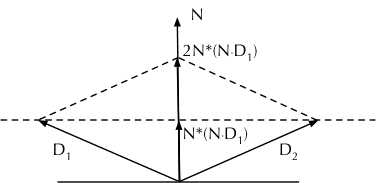} else if (obj.refl == SPEC)            // Ideal SPECULAR reflection
return obj.e + f.mult(radiance(Ray(x,r.d-n*2*n.dot(r.d)),depth,Xi));

### 折射

\begin{equation}n_1\sin \theta_1 = n_2 \sin \theta_2\end{equation}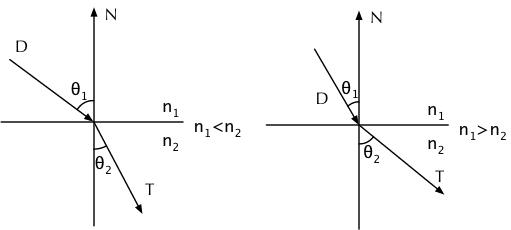#### 全反射

\begin{equation}\sin \theta_1 > \frac{n_2}{n_1} \sin(90°)\end{equation}

\begin{equation}(\frac{n_2}{n_1})^2-\cos^2 \theta_1+1<0\end{equation}

Ray reflRay(x, r.d-n*2*n.dot(r.d));     // Ideal dielectric REFRACTION
bool into = n.dot(nl)>0;                // Ray from outside going in?
double nc=1, nt=1.5, nnt=into?nc/nt:nt/nc, ddn=r.d.dot(nl), cos2t;
if ((cos2t=1-nnt*nnt*(1-ddn*ddn))<0)    // Total internal reflection
return obj.e + f.mult(radiance(reflRay,depth,Xi));

• reflRay：镜面反射方向，与上一节中的求法完全一致。
• into：由空气（试图）进入玻璃则为 true，由玻璃（试图）进入空气则为 false
• ncnt：空气和玻璃的折射率。
• nnt 为折射率比值，当追踪的射线是从空气进入玻璃时，nnt1.0/1.5，当射线是从玻璃进入空气时，nnt1.5
• ddn：即 $-\cos \theta_1$ 的值，由 $D$ 和 $N$ 点乘而来。

#### 正常折射

1. 计算折射光线矢量 $T$。
2. 计算有多少比例的光线被反射，有多少比例的光线被折射。
3. 根据条件，选择以随机或非随机的形式，递归地进行光线追踪。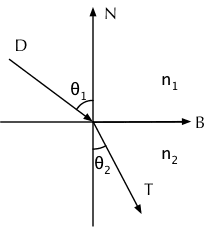\begin{equation}T=B\sin \theta_2 - N\cos \theta_2\end{equation}

\begin{equation}B=\frac{D-N\ast (D\cdot N)}{\sqrt{1-(D\cdot N)^2}}\end{equation}

\begin{equation}\sin \theta_2 = \frac{n_1}{n_2} \sin \theta_1 = \frac{n_1}{n_2} \sqrt{ 1-(D\cdot N)^2}\end{equation}

\begin{equation}\cos \theta_2 = \sqrt{1-\sin^2\theta_2} = \sqrt{1-\frac{n_1^2}{n_2^2}(1-(D\cdot N)^2)}\end{equation}

\begin{equation}T=\frac{n_1(D+N(D\cdot N))}{n_2}-N \sqrt{1-\frac{n_1^2(1-(D\cdot N)^2)}{n_2^2}}\end{equation}

Vec tdir = (r.d*nnt - n*((into?1:-1)*(ddn*nnt+sqrt(cos2t)))).norm();

\begin{equation}R_0=(\frac{n_1-n_2}{n_1+n_2})^2\end{equation}

\begin{equation}R_\theta=R_0+(1-R_0)(1-\cos \theta_1)^5\end{equation}

1. 如果深度小于等于 2，那么分别递归地追踪反射光和折射光，将两者对颜色的贡献再加上物体本身的发光，作为结果返回。
2. 如果深度大于 2，那么依据折射和反射光线的占比来进行概率随机，选择追踪反射光或折射光中的一支。换言之，如果反射光占比高，那么就有较大的可能性随机到反射光线，反之亦然。注意，这时由于我们放弃了另一种可能性，会导致结果的期望产生偏差，所以需要进行一次修正。

double a=nt-nc, b=nt+nc, R0=a*a/(b*b), c = 1-(into?-ddn:tdir.dot(n));
double Re=R0+(1-R0)*c*c*c*c*c,Tr=1-Re,P=.25+.5*Re,RP=Re/P,TP=Tr/(1-P);
return obj.e + f.mult(depth>2 ? (erand48(Xi)<P ?   // Russian roulette
radiance(reflRay,depth,Xi)*Re+radiance(Ray(x,tdir),depth,Xi)*Tr);

• ab：用以计算 $R_0$ 的临时变量。
• R0：入射角为 0 时反射光的占比。
• c：即 $1-\cos \theta_1$。
• Re：当前入射角下反射光的占比。
• Tr：折射光的占比。
• RP：随机到反射光时，对结果的修正的因子。
• TP：随机到折射光时，对结果的修正的因子。